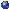## Events

##### AnalysisAndrzej Swiech, PMA 10.176: Finite dimensional approximations of Hamilton-Jacobi-Bellman equations in spaces of probability measures and stochastic optimal control of particle systems
Wednesday, March 29, 2023, 01:00pm - 02:00pm
We will discuss recent results about a class of Hamilton-Jacobi-Bellman (HJB) equations in spaces of probability measures that arise in the study of stochastic optimal control problems for systems of $n$ particles with common noise, interacting through their empirical measures. We will present a procedure to show that the value functions $u_n$ of $n$ particle problems, when converted to functions of the empirical measures, converge as $n\to\infty$ uniformly on bounded sets in the Wasserstein space of probability measures to a function $V$, which is the unique viscosity solution of the limiting HJB equation in the Wasserstein space. The limiting HJB equation is interpreted in its "lifted" form in a Hilbert space, a technique introduced by P.L. Lions. The proofs of the convergence of $u_n$ to $V$ use PDE viscosity solution techniques. An advantage of this approach is that the lifted function $U$ of $V$ is the value function of a stochastic optimal control problem in the Hilbert space. We will discuss how, using Hilbert space and classical stochastic optimal control techniques, one can show that $U$ is regular and there exists an optimal feedback control. We then characterize $V$ as the value function of a stochastic optimal control problem in the Wasserstein space. The talk will also contain an overview of existing works and various approaches to partial differential equations in abstract spaces, including spaces of probability measures and Hilbert spaces.Location: PMA 10.176